Adapted for the Internet from:Why God Doesn't Exist

1.0   The 'here and now' point

Hermann Weyl is one of the unsung authorities of relativity. His book Space, Time, Matter is essential
reading for Pastor Al's followers. Weyl begins his book by reviewing the basic geometric figures -- point,
line, plane, etc. -- which he later uses to construct and support Einstein's famous theory.

When a mathematician utters the word 'point' people imagine a dot in the horizon, an infinitesimal speck
that they can barely see. This is not what Herr Herman has in mind. Weyl begins his introduction to
Geometry by defining a point as a 'here':

" Just as we fixed the present moment ('now') as a geometrical point in time, so we fix an
exact 'here,' a point in space" (p. 11)  

Now how 'exact' can a here be if it is merely a relation to there? We're already on the wrong foot. It makes
you wonder what Weyl could possibly be visualizing and how he intends to relate this nonsense to
Geometry and to the real world.

The word 'here' is a relation between at least two things. We cannot conceive of a 'here' without a 'there'.
The word 'now' is also a relation. It implicitly invokes 'before' or 'later' or 'afterwards' or 'earlier.' We need
to listen to an explanation to understand the words 'here' or 'now'. In contrast, we need to view a single
snapshot to visualize a dot. Weyl points to a static dot and asks us to sit through a movie to 'understand'
a geometric figure. This promises to be an unforgettable experience. Unfortunately for him, in Science,
we don't understand geometric figures such as points and solids. We look at them with our eyes.
Whether the observer 'understands' the figure (i.e., processes the information in his mind) is irrelevant
to what the figure is on its own.

The mathematician may quip that my objection is cosmetic, a question of language.

It is not. Weyl later places an infinite number of these 'heres and nows' between two 'heres and nows.'
Later, he forgets his 'here and now' altogether and treats it as a geometric figure. Weyl builds lines,
constructs planes, and marks locations on solids with this 'here and now.'. He suggests that a 'here and
now' occupies a location like a chair would and that, with enough effort, you can squeeze one between
two boxes. The scholars at the Mathematical Funny Farm apparently spend their time lubricating 'nows'
and sliding them between 'here and there and everywhere' (Fig. 1) or between the past and the future
(Fig. 2). Therefore, if Weyl intends to use his idiotic concoction in Geometry or Physics or Science, this is
more than a semantic issue.
 Weyl's idiotic all-in-one line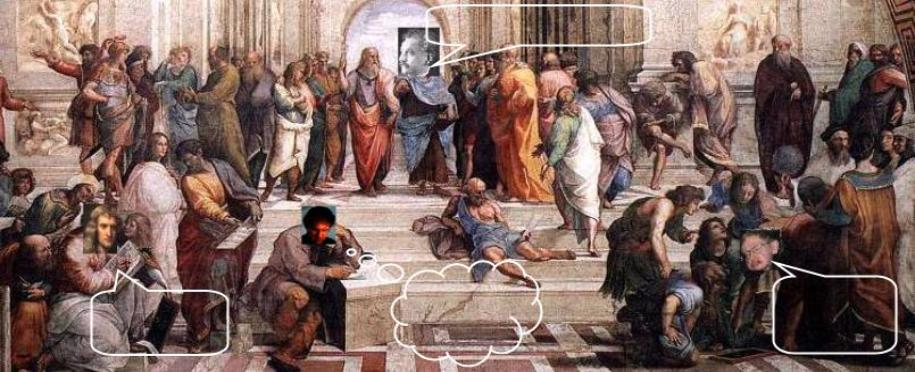A line is a point moving from here to there.
 A line is an axis of rotation.
 A line is a row of points, see?
A day at the School of Athens
 H. Weyl, Space-Time-Matter, Dover (1952)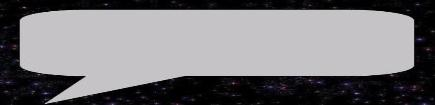Here... Now... Okay. I think I got your point, Steve.(I wonder why I must dress in silly clothes to 'understand' the point of relativity!)
 Fig. 1   Mathematical Spatial Points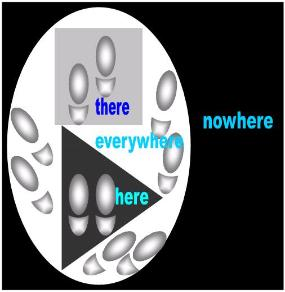Weyl proposed that a point is a 'here and now.'I think that this was a brilliant idea.  In order to distinguish the 'heres' from the 'theres' fromthe 'everywheres,' however, I would like to modify his theory a little. I propose that wemake the 'heres' like triangles, the 'theres' like squares, the 'everywheres' like circles, and the'nowheres' dimensionless.
 Fig. 2   Mathematical Temporal Points
 Likewise, I suggest that Weyl's 'now and then' points acquire some shape that will enableus to differentiate between them for the purposes of Geometry. I propose the followingconvention:
 a.       that the 'nows' henceforth be triangular  like the 'heres', the justification being that  it would thus have the same shape as a  'here'b.       the 'laters' should be like the 'theres'  and be squarishc.       the omni-present 'always' like the  omnipresent 'everywhere' should be  circulard.       the 'nevers' like the 'nowheres' should  be non-dimensionale.       the 'befores' should look like ginger-      bread men...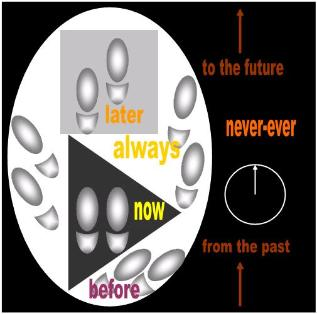Obviously, Weyl is saying in a roundabout way that a point is location. We already debunked this ludicrous
notion, so I will not repeat the arguments against it. I am more concerned for the moment with consistency.
Having just finished defining a point as a location, Weyl immediately converts it into a physical object with a
swift stroke of his magic wand:

" To every point P of S there corresponds one definite homologous point P' of S' which,
after the above displacement to a new position, would be surrounded by exactly the
same part of the given content as that which surrounded P originally... Provided that the
appropriate subjective conditions are satisfied the given material thing would seem to us
after the displacement exactly the same as before" (p. 11)

This is really getting scary now. Weyl has just moved a 'here' to over there and converted this moving
location into a material object. Think of transferring love from Sue to Peggy, and when love finally reaches
Peggy it suddenly converts into a heart. Peggy will never speak to you again!

But Weyl's magical point is just the beginning. Now comes the fun part. We get to the juicy part of his
presentation where we construct the line of Geometry with this malleable point.

2.0   Weyl's ridiculous line

Weyl has so many irreconcilable notions built into his ridiculous proposal that it is difficult to decide where
we should start to debunk it. Let's run through them quickly so that we have a good laugh at the fools of
Mathematics:

two points

" First of all the straight line. Its distinguishing feature is that it is determined by two of its
points." (p. 12)

If two points are the minimum requirements of a line, we're done. We have already defined the term
straight line. Why does Weyl need to spend the next ten pages 'constructing' a line to tell us what a
line is?

a distance between two points

" The straight line lies evenly between its points" (p. 12)

a hinge

" Any other line can…be brought into another position by a congruent transformation (the test
of straightness).” (p. 12)

" I shall here outline a preliminary argument in which not only the straight line, but also
the plane is based on a property of rotation." (p. 13)

Rotation? We need to rotate two points in order to get a line? I thought a line was a static geometric
figure. I thought Geometry was a static discipline.

a row of apples or a string of pearls

" a congruent transformation which transforms A into itself, and all points to the
right of A into points to the right of A, and all points to the left of A into points to
the left  of A, leaves every point of the straight line undisturbed." (p. 12)

" If A* is any point on g, then the plane which is erected normally to g at A* cuts  not
only g perpendicularly, but also all straight lines of the group of parallels." (p. 14)

"  we shall obtain an array of points on the straight line under construction" (p. 16)

" the end-points of all vectors OP …form a straight line" (p. 18)

" the sum total of all the end-points P of vectors…occupy fully an h-dimensional
point-configuration…The one-dimensional configuration of this type is called a
straight line" (p. 20)

These descriptions rule out any possibility that Weyl is alluding to a single traveling point. So how
does a mathematician reconcile these notions with the following:

tracks: footprints left by ONE apple

" The chief characteristic of translation is that all points are of equal importance in it, and
that the behaviour of a point during translation does not allow objective assertion to be
made about it, which could not equally well be made of any other point (this means that
the points of space for a given translation can only be distinguished by specifying each
one singly ['that one there'], whereas in the case of rotation, for example, the points on
the axis are distinguished by the property that they preserve their positions). (p. 14)

[Hopefully, Weyl is not suggesting that a 'here' or a 'position' preserves its position. For
the sake of his mental health, I hope he is saying that a dot is what preserves its position.]

" Let a be a translation which transfers the point Ao to A. This same translation will
transfer A1 to a point A2, A2 to A3, etc..." (p. 15)

" This does not yet give us the whole straight line, but only a series of equidistant
points on it" (p. 16)

a bunch of locations occupied by ONE apple

" Let a be a translation which transfers the point Ao to A." (p. 15)

" We may say that the straight line is derived from a point by an infinite repetition
of the same infinitesimal translation and its inverse." (p. 16)

" if n is a natural number (integer), a translation a/n exists which, when repeated
n times, gives a." (p. 16)

a single, continuous geometric figure

" all the points which we obtain finally fuse together into a linear continuum, in
which they become embedded, giving up their individual existences (this
description is founded on our intuition of continuity)." (p. 16)

a trough or channel plowed by ONE apple

" There is one and only one translation which transfers the arbitrary point A on g to
the arbitrary point A* on the same straight line." (p. 14)

a number line: a row of numbers

"  If we take all possible whole numbers as values of n this array will become denser in
proportion as n increases (p. 16)

Of course, if a line can be all of these irreconcilable notions at the same time and at the whim of a
mathematician, we can see how this versatile object-concept can come in handy to answer any question.
More revealing is that, after developing Math for 3000 years, the mathematicians still haven't discovered
their contradictions. That's why if you ever ask a mathematician what a line is, he will try to cover all the
bases... you know... just in case:

" a row of points suggested by a tightly stretched string, the edge of a desk, or a
flagpole, extended infinitely in both directions, is called a line."  

You forgot: 'a strip of cocaine,' 'a baseball hit hard and at a given level,' 'the crack between the buttocks,'
'what you feed a girl when you want to pick her up'...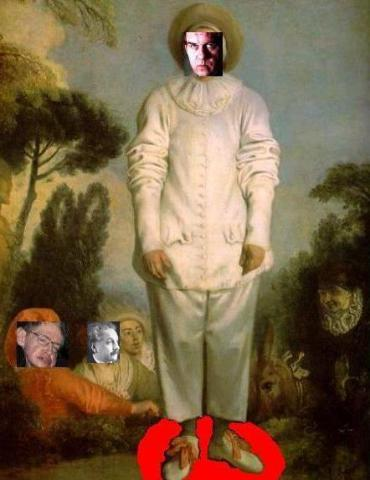________________________________________________________________________________________